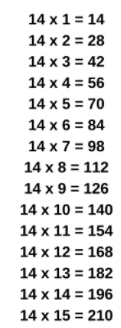# 14 Times Table- Learn Table Of 14 : Multiplication Table Of 14

Safalta expert Published by: Yashaswi More Updated Thu, 19 May 2022 10:54 PM IST

## Highlights

Check out how to learn the 14 Times table easily here at Safalta.com

Memorizing the 14 times table can be a brilliant brain-training exercise for primary-schoolers. Knowing table of 14 is a basic essential while learning mathematics. Unless you master 14 times table at the tip of your tongue, it will be difficult to perform quick mental math calculations. In this lesson, we will learn about the table of 14 with numbers from 1 to 10 along with some tricks to easily memorize these multiplication tables. Join Safalta School Online and prepare for Board Exams under the guidance of our expert faculty. Our online school aims to help students prepare for Board Exams by ensuring that students have conceptual clarity in all the subjects and are able to score their maximum in the exams.

14 Times Table Chart:## Multiplication Table of 14

Learning the Multiplication Table of 14 is necessary as it is used for long multiplication and division.

### Free Demo ClassesSource: Safalta.com

It has other advantages as well, such as:

• 14 times table helps in solving math problems easily.
• Students can use their knowledge to quickly solve any multiplication questions.
• Table of 14 helps in understanding the patterns of multiples.

Go through the 14 times table for the numbers 1 to 10 shown below.

## Tips for 14 Times Table

1. To memorize the 14 times table, first, we need to memorize the 4 times table. The multiples of 4 are 4, 8, 12, 16, 20, 24, 28, 32, 36, 40 . . .

2. To obtain the multiples of 14, add natural numbers to the tens digit of the multiples of 4. Hence, the 14 times table is obtained as follows: (1+0)4, (2+0)8, (3+1)2, (4+1)6, (5+2)0, (6+2)4, (7+2)8, (8+3)2, (9+3)6, (10+4)0 = 14, 28, 42, 56, 70, 84, 98, 112, 126, 140

3. 14 doesn’t have any rules that make the multiplication table of 14 easy to memorize, but there is a pattern for every five multiples of fourteen i.e. 14, 28, 42, 56, 70, 84, 98, 112, 126, 140. The last digit of these multiples always repeat, which means that students can remember these digits to help them with the 14 times table.

## Worksheets on 14 Times Table

1. ### Example 1: Today is Cheryl's birthday and she wants to distribute 4 candies each to her friends. If she has 14 friends in her school, using the table of 14 find how many candies would she need for distribution?

Solution:

The number of candies that Cheryl wants to distribute to each friend = 4 and the number of friends Cheryl has = 14

So, to find the total number of candies, we will determine the fourth multiple of 14.

Number of candies in all = 14 × 4 = 56.

2. ### Example 2: Using Table of 14, Find the value of 14 times 2 minus 6.

Solution:

First, we will write 14 times 2 minus 6 mathematically.

Using 14 times table, we have: 14 times 2 minus 6 = 14 × 2 - 6 = 28 - 6 = 22

Hence, 14 times 2 minus 6 is 22.

## Multiplication Tables

 2 Times Table 11 Times Table 3 Times Table 12 Times Table 4 Times Table 13 Times Table 5 Times Table 14 Times Table 6 Times Table 15 Times Table 7 Times Table 16 Times Table 8 Times Table 17 Times Table 9 Times Table 18 Times Table 10 Times Table 20 Times Table (Soon)

## Can you tell me 14 table?

Table of 14: 14 × 1 = 14, 14 × 2 = 28, 14 × 3 = 42, 14 × 4 = 56, 14 × 5 = 70, 14 × 6 = 84, 14 × 7 = 98, 14 × 8 = 112, 14 × 9 = 126, 14 × 10 = 140.

## What is the importance of learning Table 2 to 20?

For making mathematical section easier, memorising table 2 to 20 is important.

## How can I learn tables easily?

Daily recite the table as mentioned in the article twice and thrice.

## What is the times table for 14?

14 Times Table up to 10
14 × 1 = 14 14 × 6 = 84
14 × 3 = 42 14 × 8 = 112
14 × 4 = 56 14 × 9 = 126
14 × 5 = 70 14 × 10 = 140

## What is the hardest times table in the world?

The hardest multiplication was six times eight, which students got wrong 63% of the time (about two times out of three). This was closely followed by 8x6, then 11x12, 12x8 and 8x12.